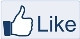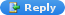# IMAGE PROCESSING

" Two roads diverged in a wood, and I,
I took the one less traveled by,
And that has made all the difference "-Robert Frost

### Oil Painting in MATLAB

To obtain a painting-like effect , define a small window matrix of size m by n. Copy the original image pixel values into the matrix and find the histogram of each value. Find the maximum occurring pixel value and replace the current position with the maximum occurrence value.Original ImageOil Paint Effect

MATLAB CODE:

%Define the matrix size of  your convience.
m=5;
n=6;
Image=uint8(zeros([size(A,1)-m,size(A,2)-n,3]));
%Calculate the histogram for each RGB value.
for v=1:3

for i=1:size(A,1)-m
for j=1:size(A,2)-n
h=zeros(1,256);
for x=1:(m*n)
end
%Maximum occurring value and the position is obtained
[maxvalue,pos]=max(h);
Image(i,j,v)=pos-1;
end
end
end
figure,imshow(Image);

SEE ALSO : Swirl effect  Cone effect    Glassy effect   Tiling effect   Paint application(coloring)
Reference:
• Algorithms for Graphics and Image Processing•  Beyond photography: The Digital Darkroom
FOR FUN:  Create your own Photoshop effectsLike "IMAGE PROCESSING" page

SADAF said...this code is working but no oil paint effect was applied
what should i do plz help :(

Aaron Angel said...Try changing the values of m and n.

SADAF said...inspite of changing the values of m and n
no change apperas in image
plz guide me wt cud be the suitable values for m and n??

SADAF said...ok i have change the values n nw its working thanx
but i have another issue
actuallyi want this code in my project where m nt using imread function rather using load funtion, which can take any user specified image at run time
then wat changes should i made to this code to set path of image??
m beginner in matlab n want dis help for my final project
plz help :(